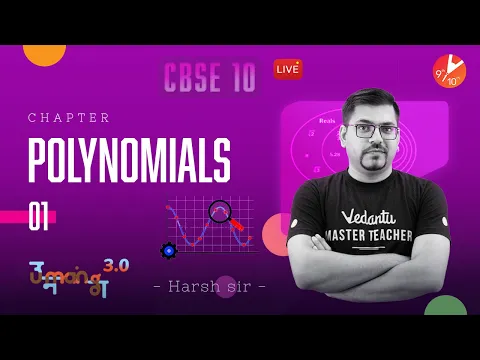Courses
Courses for Kids
Free study material
Offline Centres
MoreLast updated date: 25th Nov 2023
Total views: 629.7k
Views today: 13.29k

## NCERT Books for Class 10 Maths Chapter 2 - Polynomials - Free PDF Download

Free NCERT Books download for CBSE Class 10 Maths Chapter 2 - Polynomials on Vedantu.com. Students can also download the NCERT Textbooks Solutions in PDF for Class 10 Maths. Register for Maths tuition to clear your doubts and score more in your exams. Vedantu is a platform that provides free CBSE Solutions and other study materials for students. Subjects like Science, Maths, English will become easy to study if you have access to Class 10 Science NCERT Solutions, Maths solutions and solutions of other subjects.

Question: How to download Class 10 Maths Chapter 2 NCERT Book for CBSE?

Watch videos onSprint X Term 1 - Polynomials | NCERT Solutions Class 10 Maths Ch 2 | Board Exam 2021 Preparation
Vedantu 9&10
SubscribeShare
3.3K likes
55.8K Views
2 years agoCBSE Class 10 Maths Chapter 2 : Polynomials in One Shot Harsh Sir @VedantuClass910
Vedantu 9&10
7.5K likes
161.8K Views
2 years agoCBSE Class 10 Polynomials: Exploring Geometric Meaning & Coefficient-Root Relationship Harsh SIr
Vedantu 9&10
6.5K likes
150.9K Views
2 years agoPolynomials in One Shot | CBSE Class 10 Maths Chapter 2 NCERT Solutions | Board Exam 2021 | Vedantu
Vedantu 9&10
5.9K likes
172.9K Views
3 years ago
Play Quiz
See More### Related Chapters

More Free Study Material for PolynomialsNcert solutionsRevision notesImportant questions

## Class 10 Maths Chapter 2 - Polynomials

In this chapter in there are 3 exercise namely:

In the first exercise that is exercise 2.1, we mainly deal with zeros of the polynomial. Zeros are nothing but the values of unknown variables which satisfies the given equation. It also includes the zeros (roots) and its relationship with coefficients of the equation of quadratic polynomial.

In exercise 2.2 we deal with roots of cubic polynomials and also its relationship with the coefficients of the equations. A cubic equation has 3 roots unlike a quadratic which has 2 roots. A linear equation has only one root.

In exercise 2.3 we have division algorithms of a cubic equation and its reminder property.

### NCERT Book For Class 10 Maths

The NCERT textbooks are the prime picks for the CBSE Class 10 Mathematics. These help the students to understand the concepts in a simple and easy way. The students of CBSE Class 10 are advised to refer to and learn from the Maths Book of NCERT as it covers the entire syllabus of the CBSE.

### Major Insights to Chapter 2 Polynomials

The NCERT Class 10 Maths offers the students with some major insights including:

1. The subject experts have curated the solutions to present an easy and more relevant study guide for the students based on the questions in the books for polynomials.

2. All the key concepts are covered in the NCERT Books for polynomials to help the students to gain an in-depth understanding and knowledge of the subject.

4. The NCERT Book for class 11 maths for polynomials  is an apt resource for revision purposes and an excellent time-saver for the students.

This chapter talks about Introduction to Polynomials Geometrical Meaning of the Zeros of Polynomial Relationship between Zeros and Coefficients of a Polynomial Division Algorithm for Polynomials

### Benefits of CBSE Class 10 Maths Chapter-2

To ace the Mathematics exam for Class 10th NCERT book for Maths provides the following advantages:

• The NCERT Class 10 Maths books help the students to prepare well for school as well as competitive exams.

• The NCERT textbooks are published by experts to provide the students with authentic information about the subjects.

• The book comprises a set of questions that build a strong foundation for the concepts part.

• The NCERT book for class 10 provides a wide range of illustrative problems and solutions for the students.

• It is advisable for the students to brush up on the fundamentals from the NCERT Books of previous classes.

## FAQs on NCERT Books Free Download for Class 10 Maths Chapter 2

1. Is it useful to read the NCERT Book for Class 10 Maths?

The chapters of CBSE Class 10 are framed keeping in mind the objectives of the NCERT and are published in the NCERT Maths Books PDF. In case the students have any queries, they can refer to the NCERT Books for Class 10 available on Vedantu’s website. This will help the students to score well in their upcoming examination as this helps to simplify the complex topics and formulas of Class 10 Maths. By referring to the NCERT book the students will:

• Get more knowledge about the topics covered in Class 10 Mathematics

• Help during revision time

• Help to self-evaluate their knowledge gap using these books and prepare accordingly

• Increase their knowledge about the chief topics

• The homework and assignments given are based on these books of ease in solving them.